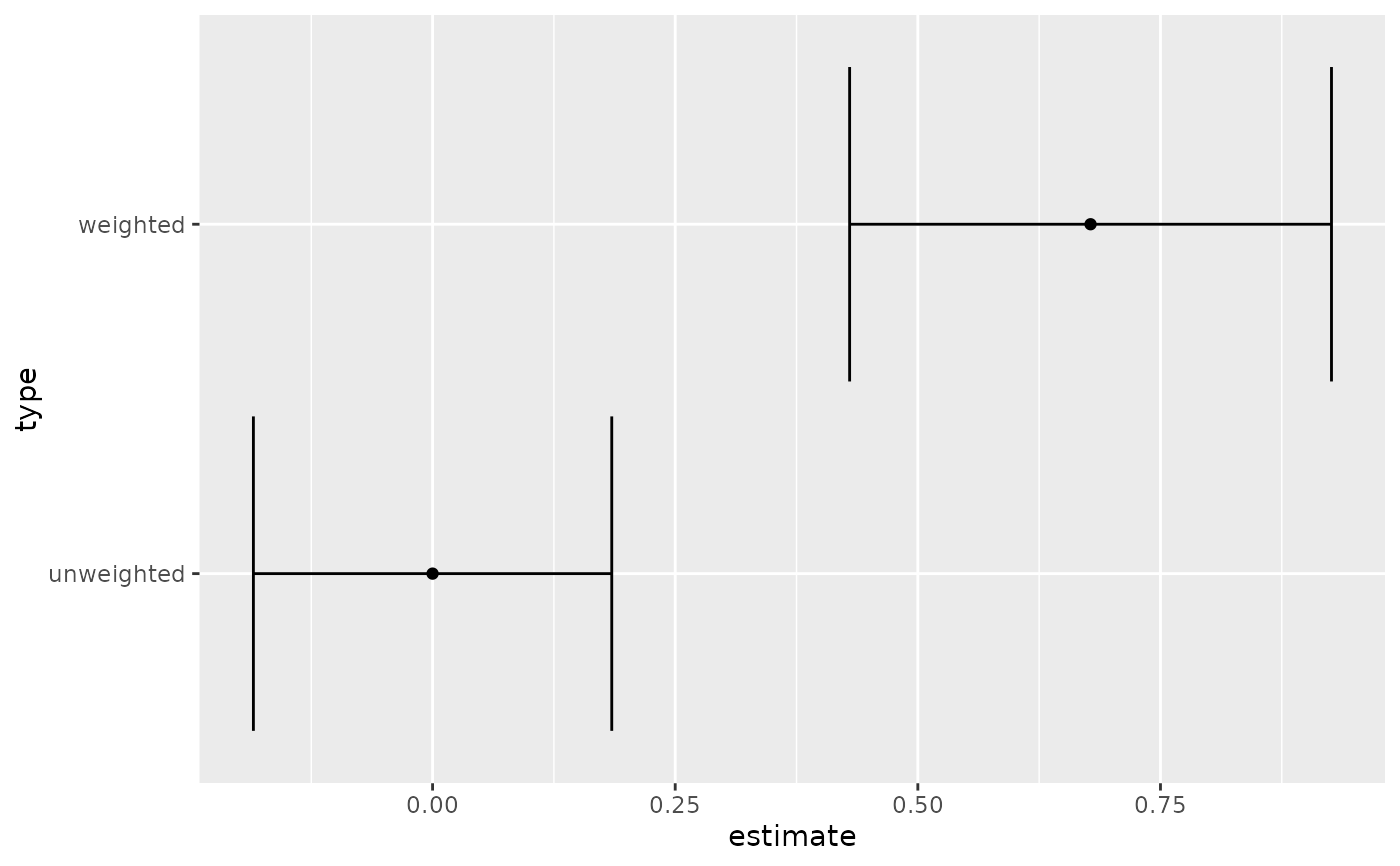Tidy summarizes information about the components of a model. A model component might be a single term in a regression, a single hypothesis, a cluster, or a class. Exactly what tidy considers to be a model component varies across models but is usually self-evident. If a model has several distinct types of components, you will need to specify which components to return.

## Usage

# S3 method for kappa
tidy(x, ...)

## Arguments

x

A kappa object returned from psych::cohen.kappa().

...

Additional arguments. Not used. Needed to match generic signature only. Cautionary note: Misspelled arguments will be absorbed in ..., where they will be ignored. If the misspelled argument has a default value, the default value will be used. For example, if you pass conf.lvel = 0.9, all computation will proceed using conf.level = 0.95. Two exceptions here are:

• tidy() methods will warn when supplied an exponentiate argument if it will be ignored.

• augment() methods will warn when supplied a newdata argument if it will be ignored.

## Details

Note that confidence level (alpha) for the confidence interval cannot be set in tidy. Instead you must set the alpha argument to psych::cohen.kappa() when creating the kappa object.

tidy(), psych::cohen.kappa()

## Value

A tibble::tibble() with columns:

conf.high

Upper bound on the confidence interval for the estimate.

conf.low

Lower bound on the confidence interval for the estimate.

estimate

The estimated value of the regression term.

type

Either weighted or unweighted.

## Examples


# load libraries for models and data
library(psych)
#>
#> Attaching package: ‘psych’
#> The following object is masked from ‘package:boot’:
#>
#>     logit
#> The following object is masked from ‘package:lavaan’:
#>
#>     cor2cov
#> The following object is masked from ‘package:car’:
#>
#>     logit
#> The following object is masked from ‘package:drc’:
#>
#>     logistic
#> The following objects are masked from ‘package:ggplot2’:
#>
#>     %+%, alpha
#> The following object is masked from ‘package:mclust’:
#>
#>     sim

# generate example data
rater1 <- 1:9
rater2 <- c(1, 3, 1, 6, 1, 5, 5, 6, 7)

# fit model
ck <- cohen.kappa(cbind(rater1, rater2))

# summarize model fit with tidiers + visualization
tidy(ck)
#> # A tibble: 2 × 4
#>   type       estimate conf.low conf.high
#>   <chr>         <dbl>    <dbl>     <dbl>
#> 1 unweighted    0       -0.185     0.185
#> 2 weighted      0.678    0.430     0.926

# graph the confidence intervals
library(ggplot2)

ggplot(tidy(ck), aes(estimate, type)) +
geom_point() +
geom_errorbarh(aes(xmin = conf.low, xmax = conf.high))